# Mechanics: Rotational Dynamics

## Rotational Dynamics: Problem Set Overview

There are 8 ready-to-use problem sets on the topic of Rotational Dynamics. These problem sets focus on the analysis of situations involving a rigid object or objects rotating in either a clockwise or counterclockwise direction about a given point. The object's rotation speed may be increasing, decreasing, or remaining constant.

## Derivation of Net Torque Equation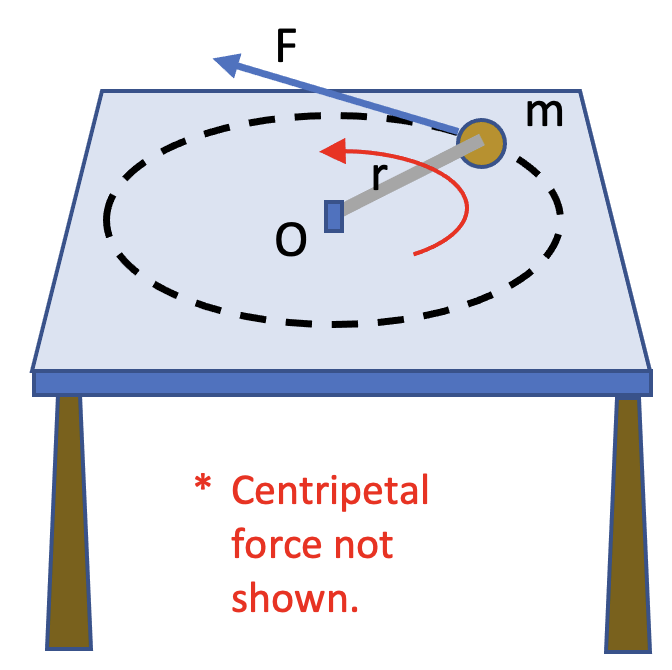Consider an object of mass, m, on a horizontal surface connected by a massless rod to a center point O. A force, F, tangent to a circle of radius, r, is applied to the mass without frictional effects as shown at right.

* We know that a circular motion requires an inward or centripetal force. In this derivation, our focus is on the effect of the tangential force. Since the centripetal force does not affect tangential force, we are not including it in this discussion.

Since the motion we care about is in the horizontal plane we can ignore the gravitational and normal force on the mass. The equation using Newton’s 2nd Law in the tangential direction would be F = m•a.

Therefore,

Ftan = m • atan.

Multiplying both sides by r:

Ftan • r = m • r •atan.

Substituting from Rotational Kinematics: atan = r • α.

Using the definition for torque: τ = r x F , which is just the left side then,

τ = m • r• α

This reveals that the torque on an object is directly related to the angular acceleration of the object, τ • a , with the constant of proportionality being m • r2. This constant of proportionality can also be thought of as the resistance to angular acceleration. This constant of proportionality or resistance to a change in motion is called the Moment of Inertia, I.

The final equation is

τ = I • α

where I = m • r2 for an object (considered a point mass) going around a center point at a constant radius.

## Moment of Inertia for Other Objects

The question arises as to what the moment of inertia, I, would be if all of the mass wasn’t concentrated at the same radius. Let’s use a solid disk and consider it to be made up of many point masses at many different radii as shown in the image below.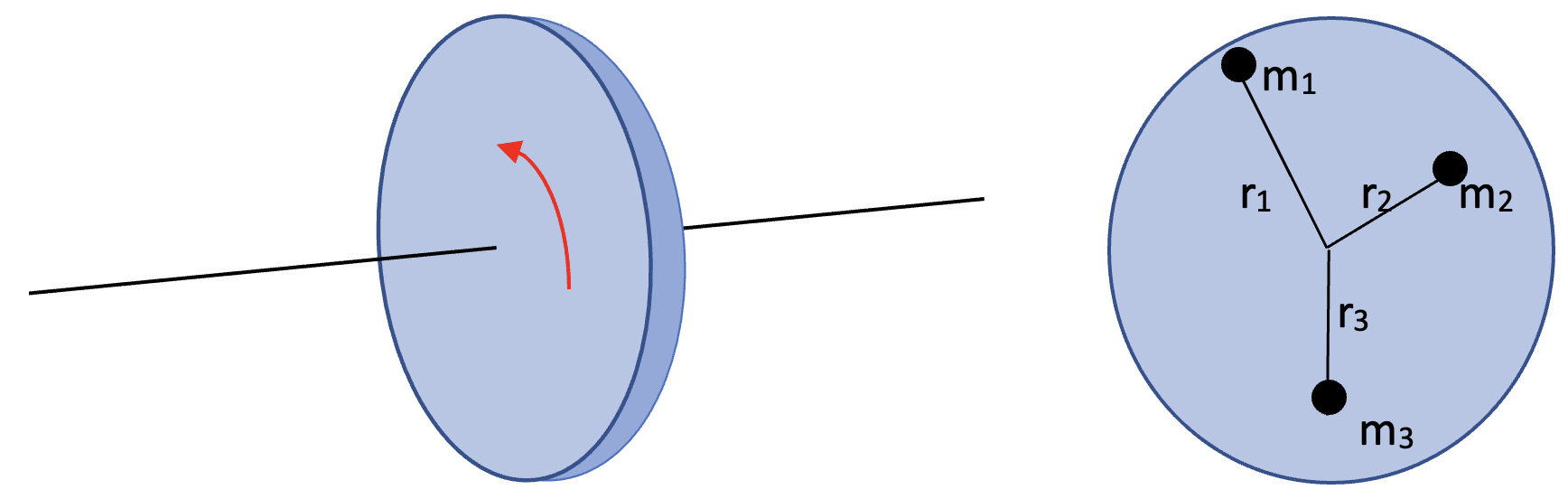The net torque on the disk is given by the sum of the individual torques on all the particles: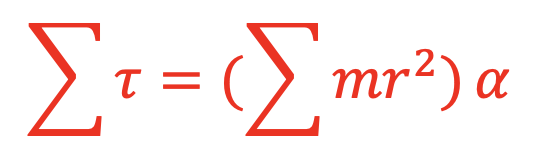Since all of the mass particles have the same angular acceleration the moment of inertia for a solid disk is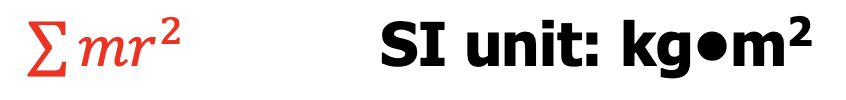Using calculus, not shown here, the moment of inertia for a solid disk of radius, R, and mass, M, isMoments of inertia for common objects of mass, M, without proof are listed below.

Object Drawing I
Hoop or
Thin Cylindrical ShellThickness of the ring is small

### M•R2

Solid Cylinder
or Disk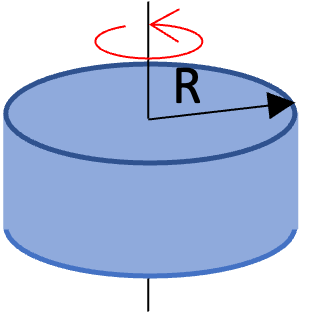### ½•M•R2

Long Thin Rod
(rotation axis
through center)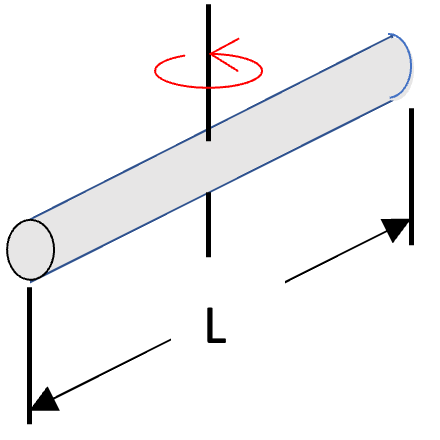### 1⁄12•M•L2

Long Thin Rod
(rotation axis
through end)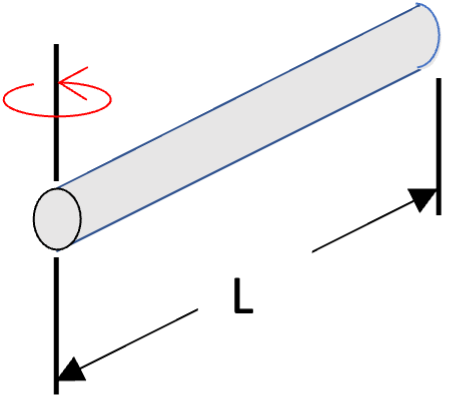### ⅓•M•L2

Solid Sphere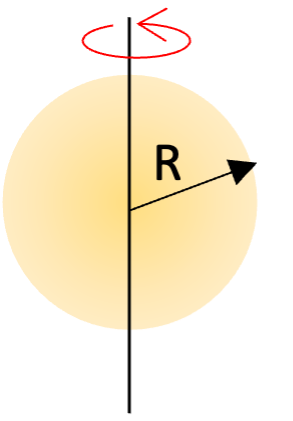### ⅖•M•R2

Thin Spherical Shell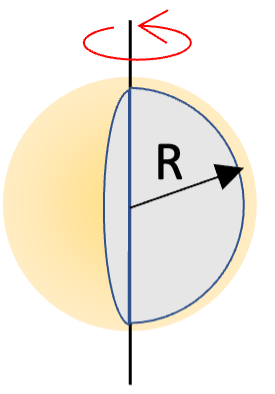Thickness of the shell is small

## Moment of Inertia for Composite Objects

Consider an obectobject that is made up of more fundamental objects as depicted in the previous table. For example, let’s look at a baton that is twirled in the middle consisting of a thin rod (mass M), and a point mass, m, on each end as shown below.The total resistance to rotation around the vertical center line has to include the rod and the 2 point masses to get the total Moment of Inertia for the system.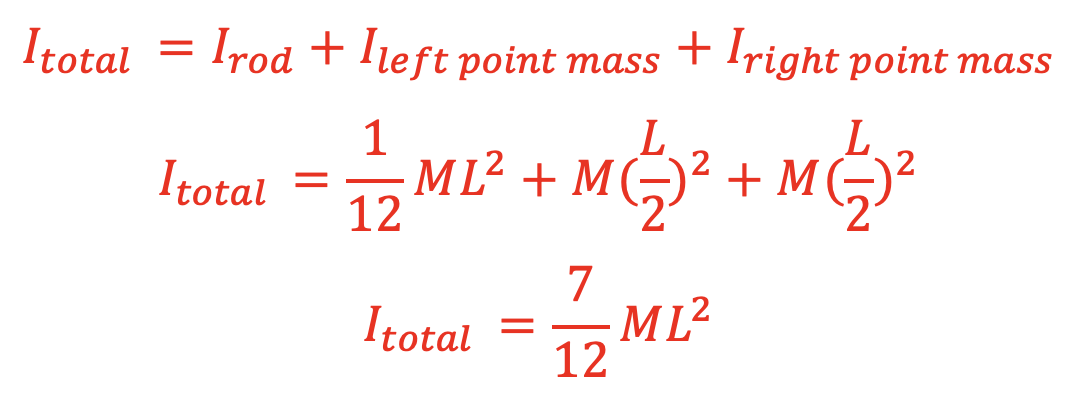## Moments of Inertia about an Unusual Axis

Consider an object that is rotating around an axis that does not go through the center of mass of the object. An example would be twirling a long, thin rod around an axis that is ¼ of the way from the center of mass. To determine this Moment of Inertia we use the Parallel Axis Theorem that states:

The Moment of Inertia of an object of mass, M, about an axis a distance, d, from the center of mass and parallel to an axis going through the center of mass is calculated by the following:

####Let’s use the long, thin rod as shown below to calculate I about the axis through Point B. The axis through Point A is going through the center of mass.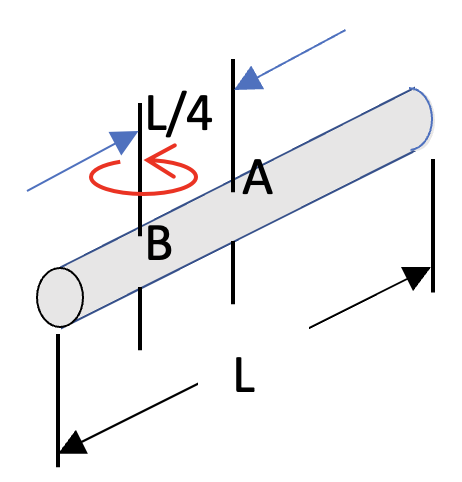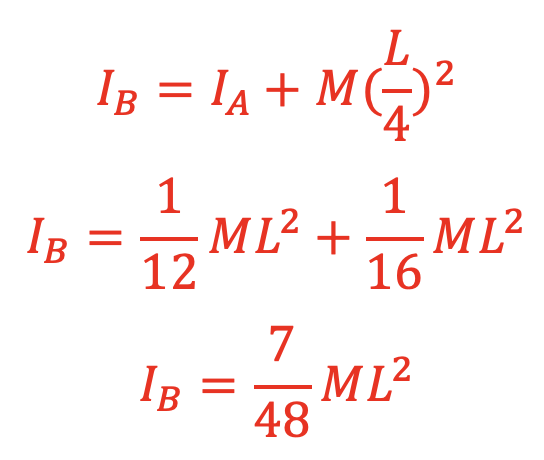## Rotational Dynamics Equations

We can now combine the Angular and Linear Kinematics Equations with the Cause of Acceleration Equations.## Derivation of Rotational Kinetic EnergyConsider an object of mass, m, on a horizontal surface connected by a massless rod to a center point O. The mass has a constant speed in the tangential direction to a circle of radius, r.

In solving Mechanics problems it can be advantageous to analyze motion using an Energy approach. The Kinetic Energy of the mass would be as shown below:

KE = ½•m•vtan2

Since vtan = r*ω, this could be written as

KE = ½•m•r2•ω2

And since I = m•r2 for a point mass the Kinetic Energy of this mass rotating around a center point could be written as
KE = ½•I•ω2What if the object was more than just a single point mass? Consider the 3 different point masses shown in the rotating solid disk around Point O at right. In order to determine their total Kinetic Energy you would have to sum them up individually as shown below:
KE = ½•m1•v12 +  ½•m2•v22 +  ½•m3•v32

Since all point masses have the same rotational speed, ω, and since v = r•ω, this could be written as
KE = ½•m1•r12•ω12 +  ½•m2•r22•ω​22 +  ½•m3•r32•ω​32

KE = ½•(∑m•r2)•ω2

If i particles in the disk are considered as i goes to infinity then ∑m•r2 = Idisk. The Kinetic Energy for the disk can then be written as
KE = ½•Idisk•ω2

The Kinetic Energy for any other object rotating around an axis with rotational speed, ω, and moment of inertia, I, can be written as
KE = ½•I•ω2

## Kinetic Energy of Rolling Objects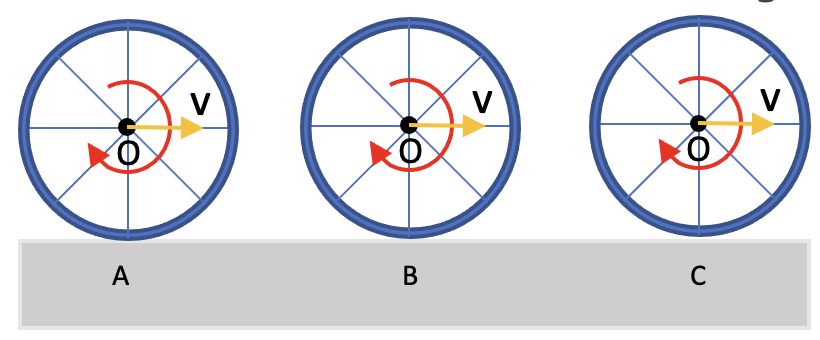How would you determine the Kinetic Energy for an object that is rotating and translating? Let’s look at the ring moving from Position A to Position C as it rolls along the surface as shown at right. It has rotational speed, ω, around Point O, horizontal center of mass speed, v, and radius, r.

Let’s analyze the motion from the center of mass position, Point O. There would be the rotational Kinetic Energy, and since point O is moving relative to the horizontal surface, there would have to be translational Kinetic Energy as well: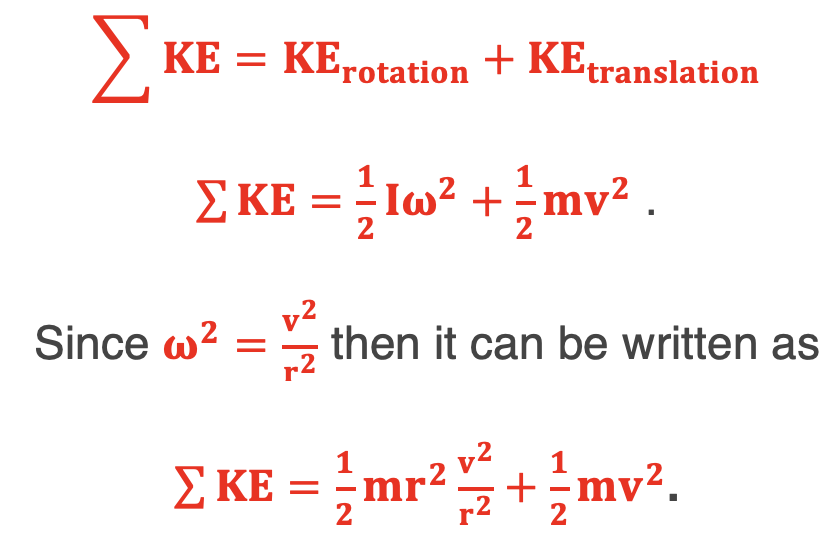Therefore, the total (translational plus rotational) Kinetic Energy for the rolling ring is KE = m•v2.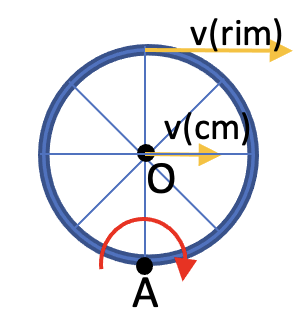A way to check that result would be to calculate the rotational Kinetic Energy around a point of contact between the ring and the horizontal surface, Point A, as shown at right. This will result in the rotational speed, ω, around Point A as depicted by the red arrow.

In this case, since the axis of rotation is not through the center of mass, Point O, the parallel axis thereom is used to determine IA. That would be

IA = IO + m•d2 = m•r2 + m•r2 = 2•m•r2

The total Kinetic Energy would be rotational only as the ring is rotating around point A at this instant and not translating (the point of contact on the ring is not moving relative to Point A on the horizontal surface).

Therefore, KE = ½•IA•ω2. and since ω = vcm/r, then

KE = ½•(2•m•r2)•(vcm/r)2

vcm relative to Point A in this case is the same as the previous horizontal center of mass speed, v, relative to the horizontal surface. In both cases then the answer to the original question (How would you determine the Kinetic Energy for an object that is rotating and translating?) is

KE = m•v2.

## Angular Momentum

In the linear case momentum (p = m•v) was used to analyze objects in motion. When doing that it was necessary to determine if the system experienced a net external force that would cause a change in momentum. If the system did not experience a net external force the system was said to be isolated resulting in the total momentum of the system being conserved (∑pinitial = ∑pfinal).

In a similar way angular momentum can be used to analyze objects rotating around an axis. Angular momentum, L,  can be defined as L = I•ω. Consider the definitions for torque and angular acceleration;then using substitution to getand finally multiplying each side by ∆t giving the resultThus, a change in angular momentum occurs if a net external torque acts on the system over a period of time. If the net external torque = 0, then the system is said to be isolated resulting in the total angular momentum of the system being conserved (∑Linitial = ∑Lfinal), similar to the case in the linear situation.

## Particle Angular MomentumConsider an object of mass, m, on a horizontal surface connected by a massless rod to a center point O. The mass has a constant speed in the tangentinal direction to a circle of radius, r. The angular momentum of the point mass would be

L = I•ω

Substituting for I and ω gives

L = m•r2•(vtan/r) = m•r•vtan

This is the magnitude of the vector cross product for the angular momentum of a particle about the vertical axis through Point O;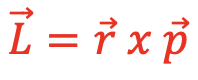To see how to calculate a value of the angular momentum vector in general for a point mass let’s consider the point mass below having a momentum, p, with an axis of rotation through point A (this will be a line in and out of the page). Since the linear momentum, p, does not lie on a line of action passing through point A it will produce an angular momentum about that point at this instant.The evaluation of the vector cross product using r and p in an algebraic calculation is shown below left. The relationship of r, p, andrelative to point A is shown below right.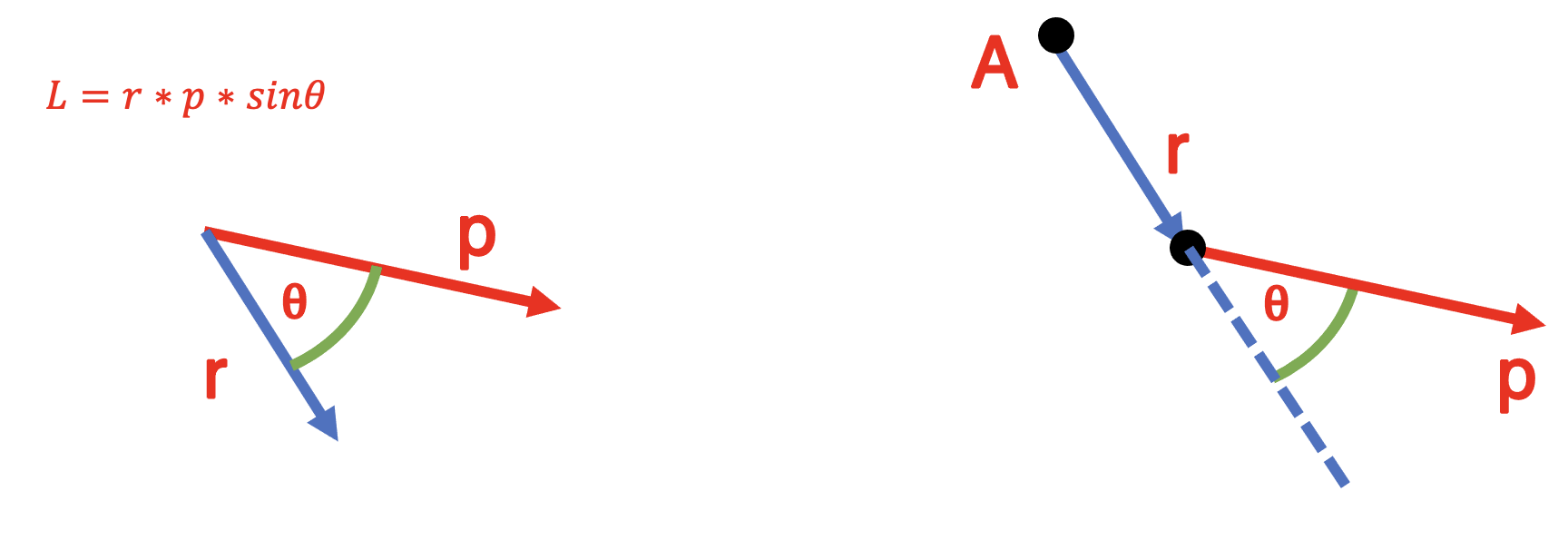Therefore, the angular momentum at this instant would evaluate to

Lpoint mass = r•m•v•sinθ.

## Rotational Dynamics Equations for Momentum

We can now combine the linear momentum case and the angular momentum case.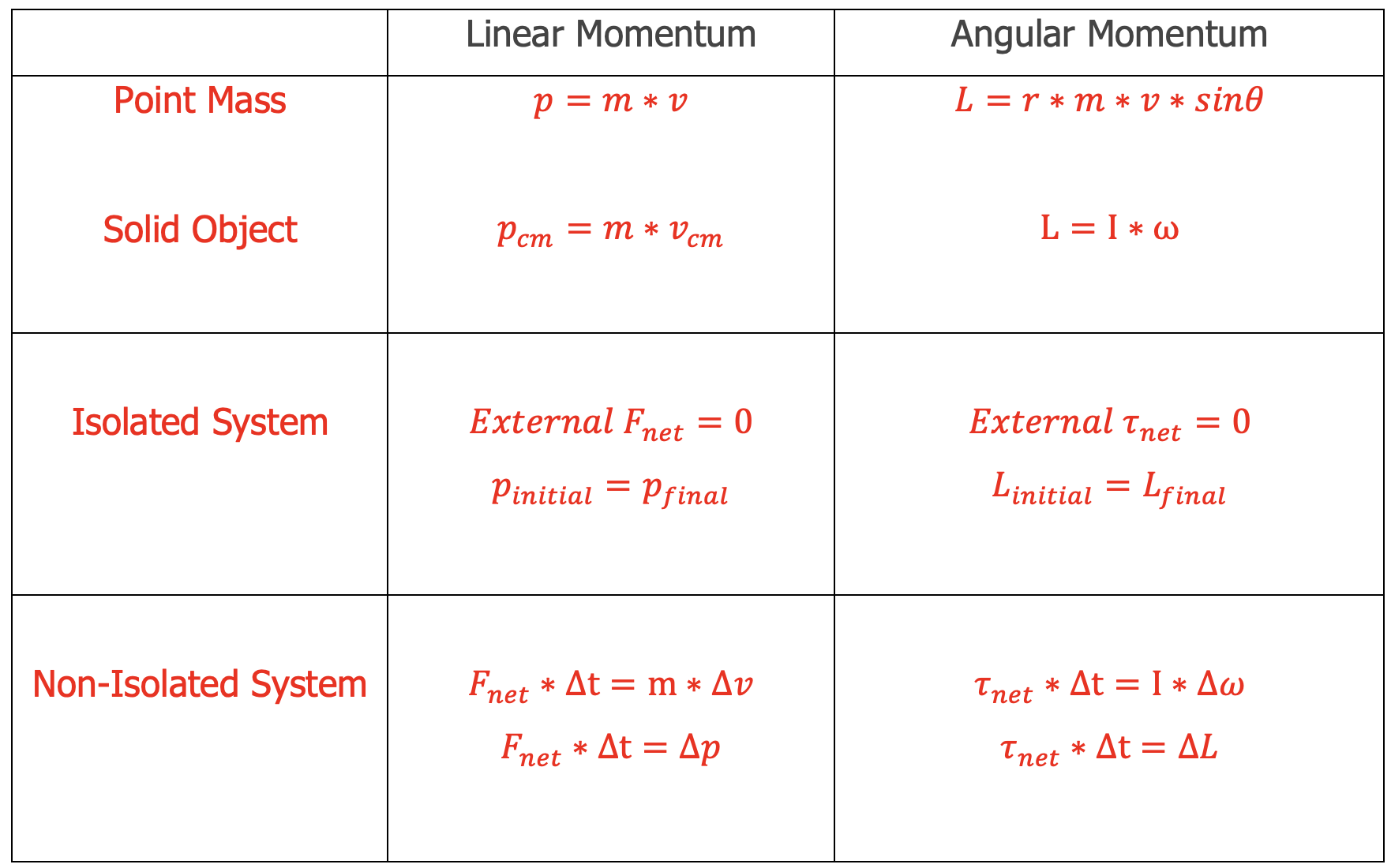## Summary of Conditions for Rotational Dynamics

One difficulty a student may encounter with this topic is the confusion as to which formula to use. When approaching these problems, it is suggested that you practice the usual habits of an effective problem-solver; identify known and unknown quantities in the form of the symbols of physics formulas, plot out a strategy for using the knowns to solve for the unknown, and then finally perform the necessary algebraic steps and substitutions required for the solution.

## Habits of an Effective Problem-Solver

An effective problem solver by habit approaches a physics problem in a manner that reflects a collection of disciplined habits. While not every effective problem solver employs the same approach, they all have habits which they share in common. These habits are described briefly here. An effective problem-solver...

• ...reads the problem carefully and develops a mental picture of the physical situation. If needed, they sketch a simple diagram of the physical situation to help visualize it.
• ...identifies the known and unknown quantities in an organized manner, often times recording them on the diagram itself. They equate given values to the symbols used to represent the corresponding quantity (e.g., m = 1.50 kg, ,  F = 12.2 N, r = 0.133 m, Torque = ???).
• ...plots a strategy for solving for the unknown quantity. The strategy will typically center saround the use of physics equations and is heavily dependent upon an understanding of physics principles.
• ...identifies the appropriate formula(s) to use, often times writing them down. Where needed, they perform the needed conversion of quantities into the proper unit.
• ...performs substitutions and algebraic manipulations in order to solve for the unknown quantity.